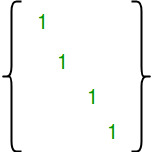# Number of Reflexive Relations on a Set

Given a number n, find out number of Reflexive Relation on a set of first n natural numbers {1, 2, ..n}.

Examples :

```Input  : n = 2
Output : 4
The given set A = {1, 2}. The following
are reflexive relations on A * A :
{{1, 1), (2, 2)}
{(1, 1), (2, 2), (1, 2)}
{(1, 1), (2, 2), (1, 2), (2, 1)}
{(1, 1), (2, 2), (2, 1)}

Input  : n = 3
Output : 64
The given set is {1, 2, 3}. There are
64 reflexive relations on A * A :
```

Explanation :

Reflexive Relation : A Relation R on A a set A is said to be Reflexive if xRx for every element of x ? A.

The number of reflexive relations on an n-element set is 2n2 – n

How does this formula work?
A relation R is reflexive if the matrix diagonal elements are 1.If we take a closer look the matrix, we can notice that the size of matrix is n2. The n diagonal entries are fixed. For remaining n2 – n entries, we have choice to either fill 0 or 1. So there are total 2n2 – n ways of filling the matrix.

## CPP

 `// C++ Program to count reflexive relations ` `// on a set of first n natural numbers. ` `#include ` `using` `namespace` `std; ` ` `  `int` `countReflexive(``int` `n) ` `{ ` `   ``// Return 2^(n*n - n)  ` `   ``return` `(1 << (n*n - n)); ` `} ` ` `  `int` `main() ` `{   ` `    ``int` `n = 3; ` `    ``cout << countReflexive(n); ` `    ``return` `0; ` `} `

## Java

 `// Java Program to count reflexive ` `// relations on a set of first n ` `// natural numbers. ` ` `  `import` `java.io.*; ` `import` `java.util.*; ` ` `  `class` `GFG { ` `     `  `static` `int` `countReflexive(``int` `n) ` `{ ` ` `  `// Return 2^(n*n - n)  ` `return` `(``1` `<< (n*n - n)); ` ` `  `} ` ` `  `// Driver function ` `    ``public` `static` `void` `main (String[] args) { ` `    ``int` `n = ``3``; ` `    ``System.out.println(countReflexive(n)); ` `         `  `    ``} ` `} ` ` `  `// This code is contributed by Gitanjali. `

## Python3

 `# Python3 Program to count ` `# reflexive relations ` `# on a set of first n ` `# natural numbers. ` ` `  `  `  `def` `countReflexive(n): ` ` `  `    ``# Return 2^(n*n - n)  ` `    ``return` `(``1` `<< (n``*``n ``-` `n)); ` ` `  `# driver function ` `n ``=` `3` `ans ``=` `countReflexive(n); ` `print` `(ans) ` ` `  `# This code is contributed by saloni1297 `

## C#

 `// C# Program to count reflexive ` `// relations on a set of first n ` `// natural numbers. ` `using` `System; ` ` `  `class` `GFG { ` `     `  `    ``static` `int` `countReflexive(``int` `n) ` `    ``{ ` `     `  `        ``// Return 2^(n*n - n)  ` `        ``return` `(1 << (n*n - n)); ` `         `  `    ``} ` `     `  `    ``// Driver function ` `    ``public` `static` `void` `Main () { ` `         `  `    ``int` `n = 3; ` `    ``Console.WriteLine(countReflexive(n)); ` `    ``} ` `} ` ` `  `// This code is contributed by vt_m. `

## PHP

 ` `

Output :

```64
```

Don’t stop now and take your learning to the next level. Learn all the important concepts of Data Structures and Algorithms with the help of the most trusted course: DSA Self Paced. Become industry ready at a student-friendly price.

My Personal Notes arrow_drop_upCheck out this Author's contributed articles.

If you like GeeksforGeeks and would like to contribute, you can also write an article using contribute.geeksforgeeks.org or mail your article to contribute@geeksforgeeks.org. See your article appearing on the GeeksforGeeks main page and help other Geeks.

Please Improve this article if you find anything incorrect by clicking on the "Improve Article" button below.

Improved By : Mithun Kumar